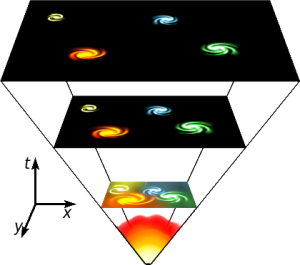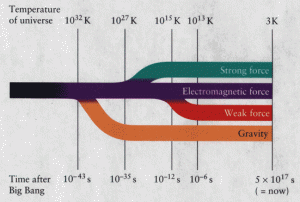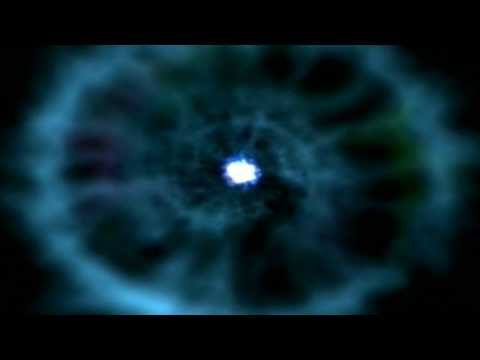Image

# The Beginning of The Universe

As we all know, the universe started from a Big Bang about 13.7 billion years ago. The Big Bang theory states that the universe started from a “singularity”, a point where space and time came into existence. This point of origin was hot and dense. Theoretically time came into existence at 5.39 * 10^-44 seconds, which is known as the Planck’s Time: the smallest unit at which time can exist. At the Planck’s time all four fundamental forces (gravity, strong force, weak force and electromagnetic force) were combined into one unified force.

Between 10^–43 seconds to 10^–36 seconds gravity separated from the unified force and this released energy. Furthermore, the strong nuclear force separated from the unified force between 10^–36 seconds to 10^–32 seconds and this triggered for the universe to go under an exponential expansion known as cosmic inflation. The following image illustrates the expansion of the
universe.This image illustrates the expansion of the universe from a singularity. Source: Wikimedia Commons

Moreover, the electromagnetic and weak forces are separated at 10^-12 seconds. The separation of the four fundamental forces released a lot of energy. The following image illustrates the separation of the four forces.One strong unified force is separated into four fundamental forces at the given times and temperatures. Source: Wikimedia Commons

The energy released form the four forces was converted to matter (Energy=mc^2 where m is mass and c is speed of light) and antimatter. Matter and antimatter was continuously created and through collisions being destroyed. At the end there was a small excess of matter over antimatter.

Then the temperatures were cooled and matter stopped being produced. The universe became a thick dense fog consisting of mostly hydrogen and some helium and lithium.

The universe is expanding even today. The most important proof for the expansion of the universe is that distant galaxies are moving away for us. The only thing expanding is space itself, the space between us and other galaxies is increasing.

The following video complements what I have stated.Naqsh Fatima Bhangu